Download Presentation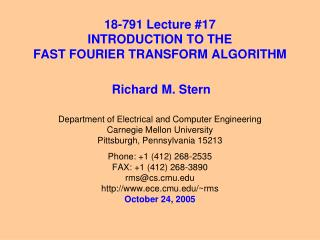18-791 Lecture #17 INTRODUCTION TO THE FAST FOURIER TRANSFORM ALGORITHM

# 18-791 Lecture #17 INTRODUCTION TO THE FAST FOURIER TRANSFORM ALGORITHM - PowerPoint PPT Presentation

18-791 Lecture #17 INTRODUCTION TO THE FAST FOURIER TRANSFORM ALGORITHM. Richard M. Stern. Department of Electrical and Computer Engineering Carnegie Mellon University Pittsburgh, Pennsylvania 15213 Phone: +1 (412) 268-2535 FAX: +1 (412) 268-3890 rms@cs.cmu.edu http://www.ece.cmu.edu/~rmsI am the owner, or an agent authorized to act on behalf of the owner, of the copyrighted work described.
Download Presentation## 18-791 Lecture #17 INTRODUCTION TO THE FAST FOURIER TRANSFORM ALGORITHM

An Image/Link below is provided (as is) to download presentation

Download Policy: Content on the Website is provided to you AS IS for your information and personal use and may not be sold / licensed / shared on other websites without getting consent from its author.While downloading, if for some reason you are not able to download a presentation, the publisher may have deleted the file from their server.

- - - - - - - - - - - - - - - - - - - - - - - - - - E N D - - - - - - - - - - - - - - - - - - - - - - - - - -
Presentation Transcript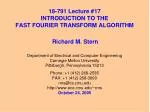### 18-791 Lecture #17INTRODUCTION TO THE FAST FOURIER TRANSFORM ALGORITHM

Richard M. Stern

Department of Electrical and Computer Engineering

Carnegie Mellon University

Pittsburgh, Pennsylvania 15213

Phone: +1 (412) 268-2535

FAX: +1 (412) 268-3890

rms@cs.cmu.edu

http://www.ece.cmu.edu/~rms

October 24, 2005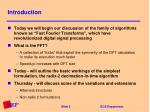Introduction
• Today we will begin our discussion of the family of algorithms known as “Fast Fourier Transforms”, which have revolutionized digital signal processing
• What is the FFT?
• A collection of “tricks” that exploit the symmetry of the DFT calculation to make its execution much faster
• Speedup increases with DFT size
• Today - will outline the basic workings of the simplest formulation, the radix-2 decimation-in-time algorithm
• Thursday - will discuss some of the variations and extensions
• Alternate structures
• Non-radix 2 formulations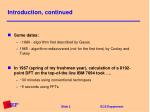Introduction, continued
• Some dates:
• ~1880 - algorithm first described by Gauss
• 1965 - algorithm rediscovered (not for the first time) by Cooley and Tukey
• In 1967 (spring of my freshman year), calculation of a 8192-point DFT on the top-of-the line IBM 7094 took ….
• ~30 minutes using conventional techniques
• ~5 seconds using FFTs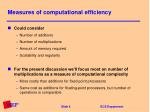Measures of computational efficiency
• Could consider
• Number of additions
• Number of multiplications
• Amount of memory required
• Scalability and regularity
• For the present discussion we’ll focus most on number of multiplications as a measure of computational complexity
• More costly than additions for fixed-point processors
• Same cost as additions for floating-point processors, but number of operations is comparable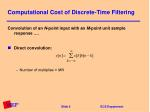Computational Cost of Discrete-Time Filtering

Convolution of an N-point input with an M-point unit sample response ….

• Direct convolution:
• Number of multiplies ≈ MN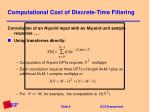Computational Cost of Discrete-Time Filtering

Convolution of an N-point input with an M-point unit sample response ….

• Using transforms directly:
• Computation of N-point DFTs requires multiplys
• Each convolution requires three DFTs of length N+M-1 plus an additional N+M-1 complex multiplys or
• For , for example, the computation is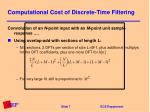Computational Cost of Discrete-Time Filtering

Convolution of an N-point input with an M-point unit sample response ….

• Using overlap-add with sections of length L:
• N/L sections, 2 DFTs per section of size L+M-1, plus additional multiplys for the DFT coefficients, plus one more DFT for
• For very large N, still is proportional to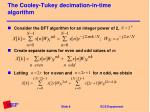The Cooley-Tukey decimation-in-time algorithm
• Consider the DFT algorithm for an integer power of 2,
• Create separate sums for even and odd values of n:
• Lettingfor n even and for n odd, we obtain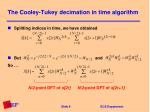The Cooley-Tukey decimation in time algorithm
• Splitting indices in time, we have obtained
• But and

So …

N/2-point DFT of x[2r]N/2-point DFT of x[2r+1]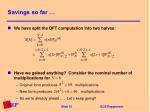Savings so far …
• We have split the DFT computation into two halves:
• Have we gained anything? Consider the nominal number of multiplications for
• Original form produces multiplications
• New form produces multiplications
• So we’re already ahead ….. Let’s keep going!!Signal flowgraph notation
• In generalizing this formulation, it is most convenient to adopt a graphic approach …
• Signal flowgraph notation describes the three basic DSP operations:
• Multiplication by a constant
• Delay

x[n]

x[n]+y[n]

y[n]

a

x[n]

ax[n]

z-1

x[n]

x[n-1]Signal flowgraph representation of 8-point DFT
• Recall that the DFT is now of the form
• The DFT in (partial) flowgraph notation:Continuing with the decomposition …
• So why not break up into additional DFTs? Let’s take the upper 4-point DFT and break it up into two 2-point DFTs:Now let’s take a closer look at the 2-point DFT
• The expression for the 2-point DFT is:
• Evaluating for we obtain

which in signal flowgraph notation looks like ...

This topology is referred to as the

basic butterflyNumber of multiplys for N-point FFTs
• Let
• (log2(N) columns)(N/2 butterflys/column)(2 mults/butterfly)

or ~ multiplysComparing processing with and without FFTs
• “Slow” DFT requires N mults; FFT requires N log2(N) mults
• Filtering using FFTs requires 3(N log2(N))+2N mults
• Let

N a1 a2

16 .25.8124

32 .156 .50

64 .0935 .297

128 .055 .171

256 .031 .097

1024 .0097 .0302

Note: 1024-point FFTs

accomplish speedups of 100

for filtering, 30 for DFTs!• As we derived it, the basic butterfly is of the form
• Since we can reducing computation by 2 by premultiplying byBit reversal of the input
• Recall the first stages of the 8-point FFT:

Consider the binary representation of the

indices of the input:

0 000

4 100

2 010

6 110

1 001

5 101

3 011

7 111

If these binary indices are

time reversed, we get the

binary sequence representing

0,1,2,3,4,5,6,7

Hence the indices of the FFT

inputs are said to be in

bit-reversed orderSome comments on bit reversal
• In the implementation of the FFT that we discussed, the input is bit reversed and the output is developed in natural order
• Some other implementations of the FFT have the input in natural order and the output bit reversed (to be described Thursday)
• In some situations it is convenient to implement filtering applications by
• Use FFTs with input in natural order, output in bit-reversed order
• Multiply frequency coefficients together (in bit-reversed order)
• Use inverse FFTs with input in bit-reversed order, output in natural order
• Computing in this fashion means we never have to compute bit reversal explicitlySummary
• We developed the structure of the basic decimation-in-time FFT
• Use of the FFT algorithm reduces the number of multiplys required to perform the DFT by a factor of more than 100 for 1024-point DFTs, with the advantage increasing with increasing DFT size
• Next time we will consider inverse FFTs, alternate forms of the FFT, and FFTs for values of DFT sizes that are not an integer power of 2